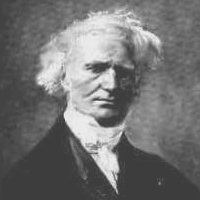# When was Matrix Multiplication invented?

Oliver Knill, June 7, 2009, last update, July 24, 2014

 To give the history of linear algebra is a task that is as important as it is difficult   -------- Nicolas Bourbaki, 1984In December 2007, Shlomo Sternberg asked me when matrix multiplication had first appeared in history. He told me about the work of Jacques Philippe Marie Binet (born February 2 1786 in Rennes and died Mai 12 1856 in Paris), who seemed to be recognized as the first to derive the rule for multiplying matrices in 1812. Shlomo also mentioned a lecture of Binet on November 30 1812 in which Binet talked about this. Here is a paper which extends Cauchy-Binet by giving a formula for the characteristic polynomial of the product of two matrices. A miniblog about this.

The question of when matrix multiplication was invented is interesting since almost all sources seem to agree that the notion of a "matrix" came only in 1857/1858 with Cayley. My own digging on Binet did not get far. It would be especially interesting to get a hand on the publication or talk of Binet of 1812 and compare it with Cauchy who would prove at the same time the Cauchy-Binet formula. Cauchy and Binet lived at around the same time, went to the same school, competed for the same jobs. Its not surprising that they discovered the Cauchy-Binet formula at around the same time. They might even have communicated about it (evenso this is only speculation).

Below are a few pointers and references. As usual in history of mathematics, one never knows for sure, when a particular structure appeared first. As for Pythagoras theorem, where Clay tablets indicate awareness of the theorem in special cases (but where Pythagoras realized first that it is a general theorem), also for determinants, there were early pre-versions. But writing down expressions like ac-bd in specific examples is not yet a general. Leibnitz first wrote down expressions like -3 5 7 + 2 6 7 + 3 4 8 - 1 6 8 - 2 4 9 + 1 5 9 indicating he understood the general structure of Sarrus rule which can be used to write down equations and understand when equations have solutions. This is why Leibnitz is often credited as the one who introduced determinants. One could distinguish three discovery points: (i) when a result is used in specific examples or applications, (ii) when it has been understood as a general rule, (iii) when it is proven. It is interesting to me that determinants have appeared before matrix algebra or even matrices and that the multiplication rule for determinants predates the discovery of matrix multiplication. But in this case one can understand the reason: Cauchy-Binet is useful when trying to understand solutions of linear equations. The later can be understood also without matrix algebra, as it happened historically.

 200 BC: Han dynasty, coefficients are written on a counting board  1545 Cardan: Cramer rule for 2x2 matrices.  1683 Seki and Leibnitz independently first appearance of Determinants  1750 Cramer (1704-1752) rule for solving systems of linear equations using determinants  1764 Bezout rule to determine determinants 1772 Laplace expansion of determinants 1801 Gauss first introduces determinants  1812 Cauchy multiplication formula of determinant. Independent of Binet 1812 Binet (1796-1856) discovered the rule det(AB) = det(A) det(B)  1826 Cauchy Uses term "tableau" for a matrix  1844 Grassman, geometry in n dimensions , (50 years ahead of its epoch [14 p. 204-205] 1850 Sylvester first use of term "matrix" (matrice=pregnant animal in old french or matrix=womb in latin as it generates determinants) 1858 Cayley matrix algebra  but still in 3 dimensions  1888 Giuseppe Peano (1858-1932) axioms of abstract vector space 
A quote from [14 p. 205] The invention of matrices illustrates once more the power and suggestiveness of a well-devised notation; it also exemplifies the fact, which some mathematicians are reluctant to admit, that a trivial notational device may be the germ of a vast theory having innumerable applications. Cayley told Tait in 1894 what led him to matrices: "I certainly did not get the notion of a matrix in any way through quaternions: it was either directly from that of a determinant; or as a convenient mode of expression of the equations
 ```x'=ax+by y' = cx+dy ```
Symbolizing this linear transformation on two independent variables by the square array
 ```|a b| |c d| ```
of its coefficients or "elements", Cayley was led to his algebra of matrices of n2 elements by the properties of linear homogeneous transformations of n independent variables.

## References

 Association J. Binet, mentions J. Shallit, Analysis of the Euclidean Algorithm, Historia Mathematica 21 (1994), 401-419.

 Grattan-Guinness, Convolutions in French Mathematics, 1800-1830 (Basel, 1990). WID-LC QA27.F8 G73 1990

 Vachov, D. Anniversaries in mathematics history for 1986. (Bulgarian) Translated in Proc. Steklov Inst. Math. 1990, no. 1, 279-284.

 Fiz.-Mat. Spis. B\cdprime lgar. Akad. Nauk. 29(62) (1987), no. 2, 118--120. Cabot Science Library PER 3740

J. Tvrdá, On the origin of the theory of matrices, Acta Historiae Rerum Naturalium necnon Technicarum (Prague, 1971), 335-354. Widener Library Info Aus 80037.5

 E. Knobloch, Der Beginn der Determinantentheorie, Leibnizens nachgelassene Studien zum Determinantenkalkül (Hildesheim, 1980).

 A.E. Malykh, Development of the general theory of determinants up to the beginning of the nineteenth century (Russian), Mathematical analysis (Leningrad, 1990), 88-97.

Peano

 Nicolas Bourbaki: Elements of the history of mathematics, 1965 Scan.

 Bell: Toward mathematical structure, Scanned pages.

 Thomas Muir, Contributions to the History of determinants, (review) Nature, 126, 839, 1930 [Scan]

 [added Aug 1, 2014] Binet's 1812 paper (Thanks to Christoph Vignat) [PDF].